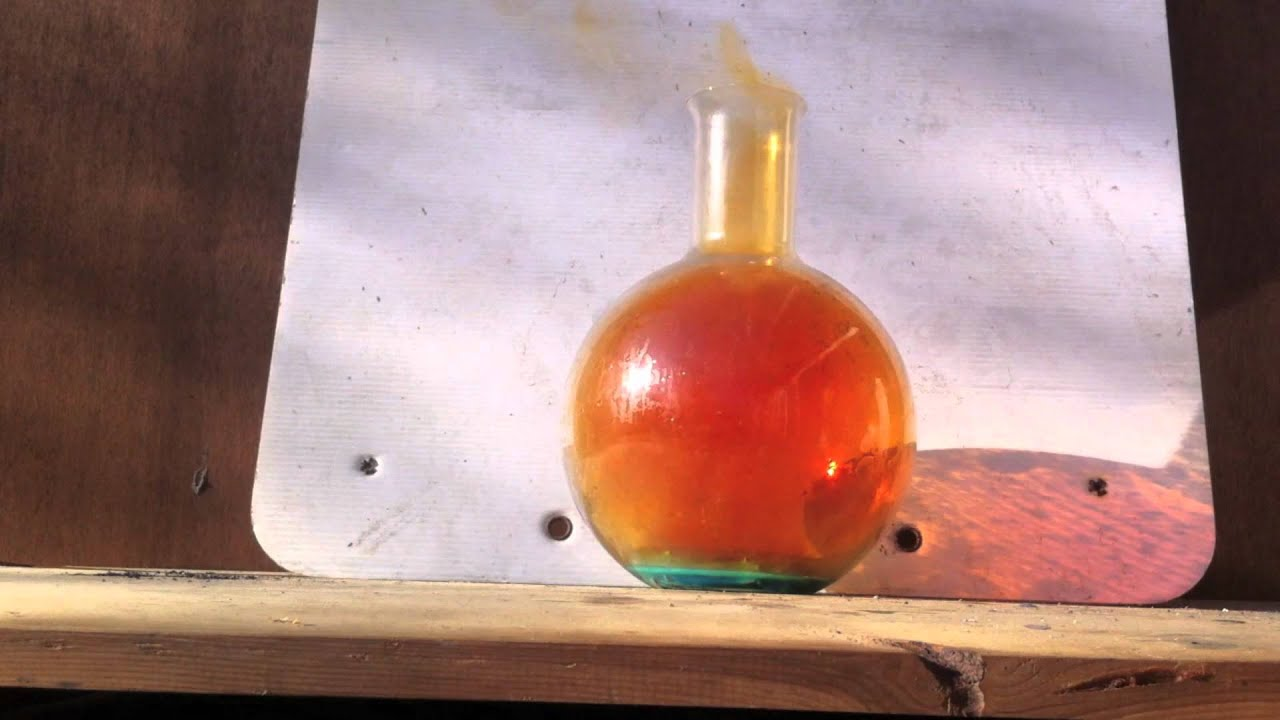# I did a Magnesium Oxide Lab.Magnesium often reacts with nitrogen in the air. If some of the magnesium had reacted with the nitrogen, would it make the % composition of ur sample higher or lower than it should be? Explain (Hint-Try a sample calculation).

##### 1 Answer
Mar 27, 2015

The % composition by mass for Mg would decrease if there was nitrogen contamination.

You would have a mixture of magnesium oxide (MgO) and a small amount of probably magnesium nitride (Mg3N2) if the sample is contaminated.

If the product is pure MgO it would be about 60% by mass Mg.

If the product was pure magnesium nitride it would be about 48% by mass Mg.

It follows that as the contamination of nitrogen increases, the % mass of Mg would decrease. This could lead you to calculate an empirical formula with subscripts different than the accepted Mg:O one:one ratio. In this case, the oxygen subscript value would be greater - you might get something like $M {g}_{8} {O}_{13}$

This formula comes from using the 48% by mass you might expect if your product was pure magnesium nitride. It is unlikely you would see much of an effect in a normal experiment.

More often, the magnesium mass is over estimated due to the presence of MgO tarnishing on the ribbon used.

If you add water to remove nitrogen contamination (done in some labs) and don't "cook" enough to evaporate it all, this shows up as a higher than actual oxygen content when you do the math. This would affect the result in the same way as nitrogen contamination.